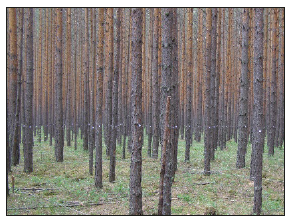• Submissions# Pinus Sylvestris L. Growth Depending on Available Resources in Ecological Niche

Alexandr N Borisov* and Viktor V Ivanov

Institute of Forest, Siberian Branch of the Russian Academy of Sciences, Russia

*Corresponding author: Alexandr N Borisov, Institute of Forest, Siberian Branch of the Russian Academy of Sciences, Russia

Submission: June 07, 2019;Published: June 13, 2019

DOI: 10.31031/EAES.2019.05.000624ISSN 2578-0336
Volume5 Issue5

#### Abstract

The technique of an estimation of spatial sharing of a resources in an ecological niche between competitors trees is offered. The technique is based on allocation of areas in which each tree has the domination in spatial sharing of a resource. The study used data from three permanent research plots even-aged pine stand based in Krasnoyarsk sporadic forest-steppe. For a studied stands close dependences of diameter of a trunk of trees and crown projection area on the area of their DA are established.

Keywords: Tree competition; Spatial sharing of a resources; Dominating area; Growth in trunk diameter; Crown projection area

#### Introduction

Modeling tree growth with regard to the available resources is an important task. Some works use competition indices based on the distance to neighbors and the size of trees . The most interesting models, in which as evaluation of available resource, there is a space of growth, or area of growth. Voronoi tessellation is used to construct polygons for each tree, formed by straight lines, which are perpendicular to the lines connecting the tree with its nearest neighbors . Voronoi polygon is formed when these perpendiculars are drawn along the middle of the distance between neighbors. The Stöhr technique uses the displacement of the sides of the polygons from the Central tree in the direction of a thinner neighbor in proportion to the diameters of their trunks . Technique Stöhr and others, used polygons for determination of the areas of growth, give their systematic overestimate for individuals of the small sizes, and understating for large individuals .

This paper consider the competition for resources based on the technique of an estimation of spatial sharing of a resources in an ecological niche. The aim of the research is to describe the impact of competition for resources on growth the diameter and the crown of pine trees by using the model of spatial sharing of a resources.

#### Discussion

The spatial model of sharing resources available to the competitor’s trees is proposed. We consider resources are factors of ecological niche required for tree growth. Competition between trees exists due to limited those resources. Borisov  assume ability trees to get resources is defined as:Where,

P(x,y)= Available resources in the point (x,y);

DBH=Diameter at breast height;

x0, y0=Coordinates of tree location;

k=Coefficient, Е0=The limit value,

If E<Е0 then ability a tree to get resources is negligible and don’t take into account.

Value Е0 defines maximal zone of influence - space of free growing. A tree space of free growing depends on DBH, soil characteristics, climate and so on . The dominating area (DA) is the area within which the tree has a dominant influence in terms of the use of available resources. For each tree DA is defined as the sum of all points in the area under consideration, where the values of E will be greater than E for all competitors. Since we use the relative values then can be put k=1. If the resources are not depend on coordinates then can be put P=1 too. The area of the DA (SDA) is taken as an estimation of the resources available for the tree.

The study used data from three permanent research plots (PRPs) even-aged pine stand based in Krasnoyarsk sporadic foreststeppe (Figure 1). Total heights and over-bark diameters at 1.3m above ground (DBH) on the PRPs were precisely measured for all trees for age of 37 and 51 years. Each PRP had at least 300 trees and the size of the each plot was 30x50 meters. The coordinates of all the trees on the PRPs were measured with a laser rangefinder with an accuracy of 10cm. The large-scale images of PRPs were made using the quadcopter DJI Phantom 3 Pro. The composition of the ground mapping data with the image was performed in ArcMap. The crowns of all trees were contoured and the areas of their projections were calculated using ArcMap.

Figure 1:The permanent research plot of even-aged pine stand of 51 age.The Figure 2 shows a composite four-layer image of a PPP fragment. The first layer is an image made by a quadrocopter, the second - white circle radii are determined by DBH and marking the positions of the trunks, the third - the contours of the crowns (marked with black lines), the fourth - the areas of domination (marked with red lines).

Figure 2:The fragment of a composite four-layer image of the PRP.The analysis of the characteristics of trunks and crowns was carried out. The obtained regression equations showed a close association DBH with SDA for unthinned PRP:

d1,3=5.63+1.94×SОД; R = 0.89,

where R=Correlation coefficient.

Regression analysis revealed the dependence of the crown projection area on the SDA:

Sкр=0.655 + 0.345×SОД; R = 0.84

#### Conclusion

A. The tree dominating area is associated with the availability of all of the required supplies for the tree growth. In forestry practice, trunk and crown size estimation is considered of vital importance because it is associated with many different factors of forest management. The process of growth of tree diameter and the formation of the crowns is determined by the competition for available resources. The contribution of the value of the available resources in the growth of trees is important for optimizing tree density for maximum productivity.

We at Crimson Publishing are a group of people with a combined passion for science and research, who wants to bring to the world a unified platform where all scientific know-how is available read more...